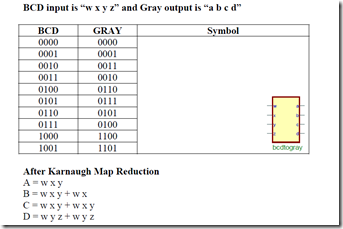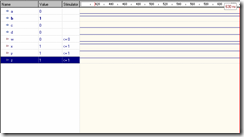# Engineering Clinic

Its all about Network Simulations, Internet of Things, Sensor Networks, Programming, etc

## Network Simulator 3

Requirements:

• WARP
• Active HDL

Procedure:

• The Specification of the BCD to GRAY Code Converter is taken.
• The input and the output ports of the above specification are defined to a Standard language (std_logic). The temporary variables are selected if necessary.
• Entity and Architecture is created for the above specification.
• The Result is verified by simulation and the waveforms are seen.

Design:Program for BCD to Gray Conversion:
library IEEE;
use IEEE.std_logic_1164.all;
entity bcdtogray is
port (
w: in STD_LOGIC;
x: in STD_LOGIC;
y: in STD_LOGIC;
z: in STD_LOGIC;
a: out STD_LOGIC;
b: out STD_LOGIC;
c: out STD_LOGIC;
d: out STD_LOGIC
);
end bcdtogray;
architecture archbcdtogray of bcdtogray is
begin
a <= w and (not x) and (not y);
b <= (w and (not x) and (not y)) or (not w and x);
c <= (not w) and (x xor y);
d <= (not w) and (y xor z);
end archbcdtogray;

Waveform